# Boundary value problems in potential theory

Fundamental problems in both classical and abstract potential theory. The classical Newton and logarithmic potentials satisfy certain partial differential equations of elliptic type: the Laplace equation in regions free of the masses generating the potentials, and the Poisson equation in regions occupied by the masses. It follows, therefore, that the boundary value problems of potential theory are primarily boundary value problems for elliptic equations and systems (cf. Boundary value problem, elliptic equations).

1) The Dirichlet problem, or first boundary value problem. Here the problem is to find a potentialin some domain, given its continuous restriction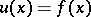,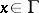, to the boundaryof the domain on the assumption that the mass distribution in the interior ofis known. This is a fundamental problem in potential theory.

2) The Neumann problem, or second boundary value problem. Here the problem is to find a potential in, given the continuous restriction of its normal derivative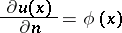to.

3) The mixed problem, or third boundary value problem. Here the known data onconstitute a linear combination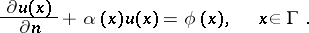(*)

4) The problem with oblique derivative arises when the normal derivativein condition (*) is replaced by the derivative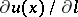with respect to an arbitrary direction,.

In addition to these general problems, the following specific problems have also arisen in potential theory.

5) The Robin problem. Here it is required to find a mass distribution onwith a constant potential in the interior of. This problem appears in electrostatics, when it is required to determine an equilibrium charge distribution on a conductorwhich has no effect anywhere inside.

6) The balayage method, which, in its simplest presentation dating back to H. Poincaré, amounts to finding a "swept-out" mass distribution onthe potential of which in the complementary domain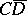coincides with the potential of given masses in the interior of. The two last problems are particularly important in abstract potential theory (see Potential theory, abstract). See also Bessel potential; Non-linear potential; Riesz potential.